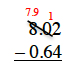Home > CC1MN > Chapter cc13 > Lesson cc13.1.2 > Problem3-35

3-35.

Find the following sums or differences. Homework Help ✎

1. $12.35 + 1.08$

• Align the terms at their decimal points.

• Add each column, starting at the right. Since $5 + 8 > 10$, carry a 1 to the next column.

• $13.43$

$\left. \begin{array} { r } { 12.35 } \\ { + 1.08 } \\ \hline \end{array} \right.$

$\left. \begin{array} { r } { 12.35 } \\ { + \quad 1.08 } \\ \hline 13.43 \end{array} \right.$

1. $8.02 − 0. 64$

Set up the problem by aligning the decimal points. Unlike in part (a), you will do subtraction for each column.

Notice that $2 < 4$, so you will need to ''borrow'' from the columns to the left.2. $568.38 − 134.21$

Use parts (a) and (b) for help.

3. $0.29 + 0.92$

Follow the steps from part (a). Remember that since $9 + 2 > 10$, you will need to carry a $1$ to the next column